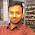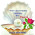## Cube and Cube Roots

###(NCERT Ex 7.1)

Q1: Which of the following numbers are not perfect cubes?
(i)  216
(ii) 128
(iii) 1000
(iv) 100
(v) 46656

(i) The prime factorisation of 216 is as follows:

 2 216 2 108 2 54 3 27 3 9 3 3 1

∴ 216 = 2 ☓ 2 ☓ 23 ☓ 3 ☓ 3 = 23 ☓ 33

Here, each prime factor appears as many times as a perfect multiple of 3, therefore, 216 is a
perfect cube.

(ii) The prime factorisation of 128 is as follows:

 2 128 2 64 2 32 2 16 2 8 2 4 2 2 1

∴  128 = 2 ☓ 2 ☓ 22 ☓ 2 ☓ 2 ☓ 2

Here, each prime factor does not appear as many times as a perfect multiple of 3. Therefore, 128 is not a perfect cube.

(iii)The prime factorisation of 1000 is as follows.

 2 1000 2 500 2 250 5 125 5 25 5 5 1

∴ 1000 = 2 ☓ 2 ☓ 25 ☓ 5 ☓ 5 =  23 ☓ 53

Here, as each prime factor appears as many times as a perfect multiple of 3, therefore, 1000 is a perfect cube.

(iv)The prime factorisation of 100 is as follows.

 2 100 2 50 5 25 5 5 1

∴ 100 = 2 ☓ 2 ☓ 5 ☓ 5

Here, each prime factor does not form triplet group(s). Therefore, 100 is not a perfect cube.

(iv) The prime factorisation of 46656 is as follows.

 2 46656 2 23328 2 11664 2 5832 2 2916 2 1458 3 729 3 243 3 81 3 27 3 9 3 3 1

46656 = 2 ☓ 2 ☓ 22 ☓ 2 ☓ 23 ☓ 3 ☓ 33 ☓ 3 ☓ 3
= 23 ☓ 2☓ 3☓ 33
Here, each prime factor appears as many times as a perfect multiple of 3,
∴ 46656 is a perfect cube.

Q2: Find the smallest number by which each of the following numbers must be multiplied to obtain a perfect cube.

(i) 243
(ii) 256
(iii) 72
(iv) 675
(v)  100

(i) 243 = 3 ☓ 3 ☓ 3☓ 3 ☓ 3

Here, two 3s are left which require one more 3 to form a triplet.
i.e. 243 ☓ 3 = 3 ☓ 3 ☓ 3 3 ☓ 3 ☓ 3 = 729 is a perfect cube.

∴ the smallest natural number by which 243 should be multiplied to make it a perfect cube is 3.

(ii) 256 = 2 ☓ 2 ☓ 2 2 ☓ 2 ☓ 2 ☓ 2 ☓ 2

Here, two 2s are left which requires one more 2 to form a triplet. To make 256 a cube, one more 2 is required.

256 ☓ 2 = 2 ☓ 2 ☓ 2 2 ☓ 2 ☓ 22 ☓ 2 ☓ 2 = 512 is a perfect cube.

Hence, the smallest natural number by which 256 should be multiplied to make it a perfect cube is 2.

(iii) 72 = 2 ☓ 2 ☓ 2 ☓ 3 ☓ 3

Here, two 3s are left which are not in a triplet. To make 72 a cube, one more 3 is required.

72 ☓ 3 = 2 ☓ 2 ☓ 23 ☓ 3 ☓ 3 = 216 is a perfect cube.

Hence, the smallest natural number by which 72 should be multiplied to make it a perfect cube is 3.

(iv) 675 = 3 ☓ 3 ☓ 3 ☓ 5 ☓ 5

Here, two 5s are left which require one more 5 to forma a triplet.

∴ 675 ☓ 5 = 3 ☓ 3 ☓ 35 ☓ 5 ☓ 5 = 3375 is a perfect cube.

Hence, the smallest natural number by which 675 should be multiplied to make it a perfect cube is 5.

(v) 100 = 2 ☓ 2 ☓ 5 ☓ 5

Here, two 2s and two 5s are left which are do not form a triplet. To make 100 a cube, we need one more 2 and one more 5.

∴ 100 ☓ 2 ☓ 5 = 2 ☓ 2 ☓ 25 ☓ 5 ☓ 5 = 1000 is a perfect cube

Hence, the smallest natural number by which 100 should be multiplied to make it a perfect cube is
2 ☓5 = 10.

Q3: Find the smallest number by which each of the following numbers must be divided to obtain a perfect cube.

(i)  81
(ii) 128
(iii) 135
(iv) 192
(v) 704

(i) 81 = 3 ☓ 3 ☓ 3 ☓ 3

Here, one 3 is left which does not form a triplet.

If we divide 81 by 3, then it will become a perfect cube.

∴ 81 ÷ 3 = 27 = 3 ☓ 3 ☓ 3 is a perfect cube.

Hence, the smallest number by which 81 should be divided to make it a perfect cube is 3.

(ii) 128 = 2 ☓ 2 ☓ 22 ☓ 2 ☓ 2 ☓ 2

Here, one 2 is left which is not in a triplet.

If we divide 128 by 2, then it will become a perfect cube.

∴ 128 ÷ 2 = 64 = 2 ☓ 2 ☓ 22 ☓ 2 ☓ 2 is a perfect cube.

Hence, the smallest number by which 128 should be divided to make it a perfect cube is 2.

(iii) 135 = 3 ☓ 3 ☓ 3 ☓ 5

Here, one 5 is left which is not part of a triplet.

If we divide 135 by 5, then it will become a perfect cube.

Thus, 135 ÷ 5 = 27 = 3 ☓ 3 ☓ 3 is a perfect cube.

Hence, the smallest number by which 135 should be divided to make it a perfect cube is 5.

(iv) 192 = 2 ☓ 2 ☓ 22 ☓ 2 ☓ 2 ☓ 3

Here, one 3 is left which is not part of a triplet.

By dividing 192 by 3, it becomes a perfect cube.

Thus, 192 ÷ 3 = 64 = 2 ☓ 2 ☓ 22 ☓ 2 ☓ 2 is a perfect cube.

Hence, the smallest number by which 192 should be divided to make it a perfect cube is 3.

(v) 704 = 2 ☓ 2 ☓ 22 ☓ 2 ☓ 2 ☓ 11

Here, one 11 is left which does not form a triplet.

If we divide 704 by 11, then it makes a perfect cube.

Thus, 704 ÷ 11 = 64 = 2 ☓ 2 ☓ 22 ☓ 2 ☓ 2 is a perfect cube.

Hence, the smallest number by which 704 should be divided to make it a perfect cube is 11.

Q4: Parikshit makes a cuboid of plasticine of sides 5 cm, 2 cm, 5 cm. How many such cuboids will he need to form a cube?

Volume of the cube of sides 5 cm, 2 cm, 5 cm = 5 cm ☓ 2 cm ☓ 5 cm = (5 ☓ 5 ☓ 2) cm3

Here, two 5s and one 2 are left which do not form a triplet.

To make it a pefect cube, multiply this expression by 2 ☓ 2 ☓ 5 = 20, then it will become a perfect cube.

i.e. (5 ☓ 5 ☓ 2 ☓ 2 ☓ 2 ☓ 5) = (5 ☓ 5 ☓ 52 ☓ 2 ☓ 2) = 1000 is a perfect cube.

Therefore 20 cuboids of 5 cm, 2 cm, 5 cm are required to form a cube.1.Go to babajikenotes.com for notes of jee main or +1 or +2

2.Go to babajikenotes.com for notes of jee main or +1 or +2

3.4.5.helped me a lot for my maths exam. you should include some extra questions too

6.7.8.